[english]
[français]

Charles Deledalle - Teaching

UCSD ECE285 Machine learning for image processing

(Spring 2019)

Lectures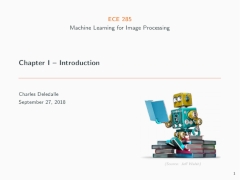Chapter 1 - Introduction (42Mb) Overview of the course. Introduction to image sciences, image processing and computer vision. Basics of machine learning, terminologies, paradigms. No-free lunch theorem. Supervised versus unsupervised learning. Clustering and K-Means. Classification and regression. Linear least squares and polynomial curve fitting. Model complexity and overfitting. Curse of dimensionality. Dimensionality reduction and principal component analysis. Image representation, semantic gap, image features, and classical computer vision pipelines.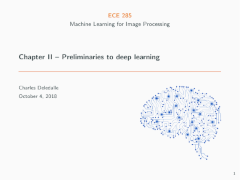Chapter 2 - Preliminaries to deep learning (13Mb) Binary classification and linear separators. Perceptron, ADALINE, artificial neurons. Artificial neural networks (ANNs), activation functions, and universal approximation theorem. Linear versus non-linear classification problems. Typical tasks, architectures and loss functions. Gradient descent and back-propagation. Support Vector Machines (SVMs), soft-margins and kernel trick. Connections between ANNs and SVMs.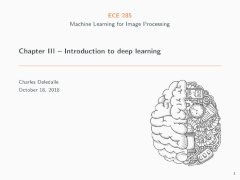Chapter 3 - Introduction to deep learning (16Mb) Feature hierarchy. Feature engineering versus end-to-end learning. Deep architectures and gradient vanishing problem. Greedy layer-wise unsupervised pretraining and stacked auto-encoders. Modern deep learning: rectifiers, gradient clipping, stochastic and mini-batch gradient descent, learning rate schedules, adaptive learning rates, momentum and Nesterov acceleration, weight decay, gradient noise, early stopping, normalized initializations, dropout, batch normalization, residual networks, curriculum learning, multi-task learning, best practice. Weakness and adversarial perturbations.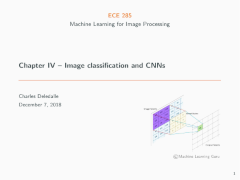Chapter 4 - Image classification and CNNs (29Mb) Linear and shift invariant feature maps, cross-correlation, convolution, boundary conditions and padding, averaging and derivative filters, filter banks, Gabor filters, and wavelets. Convolutional Neural Networks (CNNs). Hubel and Wiesel brain activity model. Simple and complex cells. Convolutional layers, pooling layers, overcomplete representation, receptive fields. Flat tensors and fully connected classification heads. Backpropagation for CNNs. Typical architectures: Neocognitron, LeNet-5, AlexNet, ZFNet, VGG, GoogLeNet, ResNet, DenseNet, MobileNet. Evaluation metrics.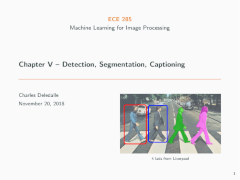Chapter 5 - Detection, Segmentation, Captioning (35Mb) Localization and classification. Overfeat: class agnostic versus class specific localization, fully convolutional neural networks, greedy merge strategy. Multi-object detection. Region proposal and selective search. R-CNN, Fast R-CNN, Faster R-CNN, R-FCN, YOLO and SSD. Image segmentation. Semantic segmentation and transposed convolutions. Instance segmentation and Mask R-CNN. Image captioning. Recurrent Neural Networks (RNNs). Language generation. Long Short Term Memory (LSTMs). DeepImageSent, Show and Tell, and Show, Attend and Tell algorithms.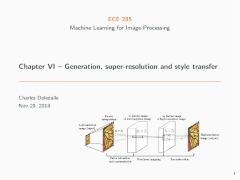Chapter 6 - Generation, Super-Resolution, Style transfer (49Mb) Image generation. Gaussian models for human faces, limits and relations with linear neural networks. Generative adversarial networks (GANs), generators, discriminators, adversarial loss and two player games. Convolutional GAN and image arithmetic. Super-resolution. Nearest-neighbor, bilinear and bicubic interpolation. Image sharpening. Linear inverse problems, Tikhonov and Total-Variation regularization. Super-Resolution CNN, VDSR, Fast SRCNN, SRGAN, perceptual, adversarial and content losses. Style transfer: Gatys model, content loss and style loss.

up

UCSD ECE285 Image and video restoration

(Spring 2019 Python version)

Lectures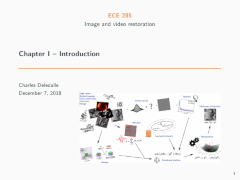Chapter 1 - Introduction (61Mb) Image sciences, image processing, image restoration, photo manipulation. Image and videos representation. Digital versus analog imagery. Quantization and sampling. Sources and models of noises in digital CCD imagery: photon, thermal and readout noises. Sources and models of blurs. Convolutions and point spread functions. Overview of other standard models, problems and tasks: salt-and-pepper and impulse noises, half toning, inpainting, super-resolution, compressed sensing, high dynamic range imagery, demosaicing. Short introduction to other types of imagery: SAR, Sonar, ultrasound, CT and MRI. Linear and ill-posed restoration problems.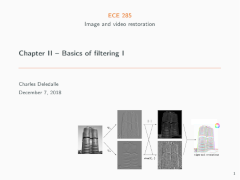Chapter 2 - Basics of filtering I: Spatial filters (25Mb) Moving averages. Finite differences and edge detectors. Gradient, Sobel and Laplacian. Linear translations invariant filters, cross-correlation and convolution. Adaptive and non-linear filters. Median filters. Morphological filters. Local versus global filters. Sigma filter. Bilateral filter. Patches and non-local means. Applications to image denoising.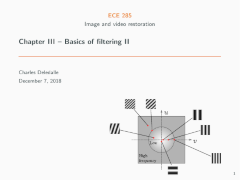Chapter 3 - Basics of filtering II: Spectral filters (17Mb) Fourier decomposition and Fourier transform. Continuous verse discrete Fourier transform. 2D Fourier transform and spectral analysis. Low-pass and high-pass filters. Convolution theorem. Image sharpening, Image resizing and sub-sampling. Aliasing, Nyquist -Shannon theorem, zero-padding, and windowing. Spectral models of sub-sampling in CT and MRI. Radon transform, k-space trajectories, and streaking artifacts.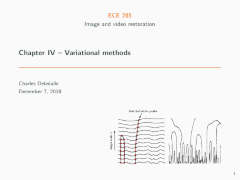Chapter 4 - Variational methods (36Mb) Heat equation. Discretization and finite difference. Explicit and implicit Euler schemes. CFL conditions. Continuous Gaussian convolution solution. Linear and non-linear scale spaces. Anisotropic diffusion. Perona-Malik and Weickert model. Variational methods. Tikhonov regularization by gradient descent. Links between variational models and diffusion models. Total-Variation regularization and ROF model. Sparsity and group sparsity. Applications to image deconvolution.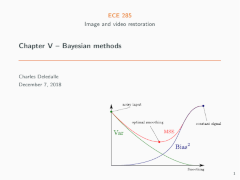Chapter 5 - Bayesian methods (58Mb) Sample mean, law of large numbers, and method of moments. Mean square error and bias-variance trade-off. Unbiased estimation: MVUE, Cramèr-Rao-Bound, Efficiency, MLE. Linear estimation: BLUE, Gauss-Markov theorem, least-square error estimator, Moore-Penrose pseudo-inverse. Bayesian estimators: likelihood and priors, MMSE and posterior mean, MAP. Linear MMSE, applications to Wiener deconvolution, image filtering with PCA, and the non-local Bayes algorithm. Applications to image denoising.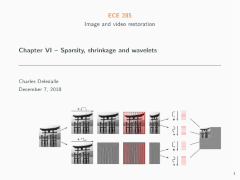Chapter 6 - Sparsity, shrinkage and wavelets (93Mb) Limits of Wiener filtering. Non-linear shrinkage functions. Limits of Fourier representation. Continuous and discrete wavelet transforms. Sparsity and shrinkage in wavelet domain. Undecimated wavelet transforms, a trous algorithm. Regularization. Sparse regression, combinatorial optimization and matching pursuit. LASSO, non-smooth optimization, and proximal minimization. Link with implicit Euler scheme. ISTA algorithm. Synthesis versus analysis regularized models. Applications to image deconvolution.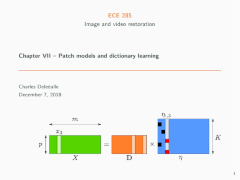Chapter 7 - Patch models and dictionary learning (34Mb) Patch models and sparse decompositions of image patches. Dictionary learning and the k-SVD algorithm. Collaborative filtering and BM3D. Non-local sparse based models. Expected patch log-likelihood. Other applications of patch models in inpainting, super-resolution and deblurring.

up

up